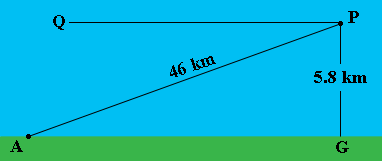SEARCH HOMEMath Central Quandaries & QueriesQuestion from Taten, a student: So you have an airplane with an altitude of 5.8KM. It has 46KM till the run way. What is the angle of decent?Hi Taten,

In my diagram $P$ is the airplane, $A$ is the airport, $G$ is the point on the ground directly below the airplane and $PQ$ is parallel to the ground.The angle of decent is the angle $QPA$ which is congruent to the angle $PAG.$ Triangle $PAG$ is a right triangle. What trig functions relates the angle $PAG$ to the lengths of the sides $PA$ and $GP?$

PennyMath Central is supported by the University of Regina and The Pacific Institute for the Mathematical Sciences.